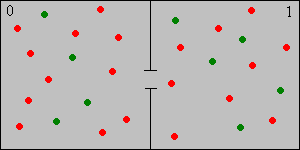]> The Bernoulli-Laplace Chain

## 7. The Bernoulli-Laplace Chain

The Bernoulli-Laplace chain, named for James Bernoulli and Pierre Simon Laplace, is a simple discrete model for the diffusion of two incompressible gases between two containers. Like the Ehrenfest chain, it can also be formulated as a simple ball and urn model. Thus, suppose that we have two urns, labeled 0 and 1. Urn 0 contains $j$ balls and urn 1 contains $k$ balls, where $j$ and $k$ are positive integers. Of the $j k$ balls, $r$ are red and the remaining $j k r$ are green. At each discrete time, independently of the past, a ball is selected at random from each urn and then the two balls are switched. The balls of different colors correspond to molecules of different types, and the urns are the containers. The incompressible property is reflected in the fact that the number of balls in each urn remains constant over time.Let $X n$ denote the number of red balls in urn 1 at time $n$. Note that

1. $k X n$ is the number of green balls in urn 1 at time $n$.
2. $r X n$ is the number of red balls in urn 0 at time $n$.
3. $j r X n$ is the number of green balls in urn 0 at time $n$.

Argue that $X X 0 X 1 X 2$ is a Markov chain on $S 0 r j k r$ with the transition probability function $P$ given below. Sketch the state graph.

$P x x 1 j r x x j k , P x x r x x j r x k x j k , P x x 1 r x k x j k ; x S$

Explicitly give the state space and the transition probability function in the following cases:

1. $j k$, so each container has the same number of particles.
2. $j k r$, so the number of particles of each type is the same, and is the number of particles in each container.

Consider the Bernoulli-Laplace chain with $j 10$, $k 5$, and $r 4$. Suppose that $X 0$ has the uniform distribution on $S$.

1. Explicitly give the transition probability function in matrix form.
2. Compute the probability density function, mean and variance of $X 1$.
3. Compute the probability density function, mean and variance of $X 2$.
4. Compute the probability density function, mean and variance of $X 3$.
5. Sketch the initial probability density function and the probability density functions in parts (a), (b), and (c) on a common set of axes.

Run the simulation of the Bernoulli-Laplace experiment for 10000 steps and for various values of the parameters. Note the limiting behavior of the proportion of time spent in each state.

#### Invariant and Limiting Distributions

Show that the Bernoulli-Laplace chain is irreducible and aperiodic.

Show that the invariant distribution is the hypergeometric distribution with population parameter $j k$, sample parameter $k$, and type parameter $r$. The probability density function is

$f x r x j k r k x j k k , x S$

Thus, the invariant distribution corresponds to selecting a sample of $k$ balls at random and without replacement from the $j k$ balls and placing them in urn 1.

Find the mean return time to each state.

Describe the limiting behavior of $P n$ as $n$.

In the simulation of the Bernoulli-Laplace experiment, vary the parameters and note the shape and location of the limiting hypergeometric distribution. For selected values of the parameters, run the simulation for 10000 steps and and note the limiting behavior of the proportion of time spent in each state.

#### Reversibility

Show that the Bernoulli-Laplace chain is reversible and use the basic reversibility condition to show again that the invariant distribution is the hypergeometric distribution with population parameter $j k$, sample parameter $k$, and type parameter $r$.

Run the simulation of the Bernoulli-Laplace experiment 10,000 time steps for selected values of the parameters, and with initial state 0. Note that at first, you can see the arrow of time. After a long period, however, the direction of time is no longer evident.